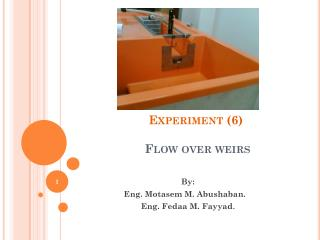# Experiment (6) Flow over weirs - PowerPoint PPT PresentationDownload PresentationExperiment (6) Flow over weirs

Experiment (6) Flow over weirs
Download Presentation## Experiment (6) Flow over weirs

- - - - - - - - - - - - - - - - - - - - - - - - - - - E N D - - - - - - - - - - - - - - - - - - - - - - - - - - -
##### Presentation Transcript

1. Experiment (6) Flow over weirs By: Eng. Motasem M. Abushaban. Eng. Fedaa M. Fayyad.

2. Introduction • Weir is defined as a barrier over which the water flows in an open channel • A weir is a notch on a larger scale – usually found in rivers. • It is used as both a flow measuring device and a device to raise water levels.

3. Introduction • Crest Is the edge or surface over which the water flows. • Nappe Is the overflowing sheet of water.

4. Introduction

5. Introduction • Usually named for the shape of the overflow opening • Rectangular • Triangular • Trapezoidal

6. Rectangular Weir

7. Triangular Weirs

8. Purpose • To observe characteristics of flow over a weir. • To determine the head-discharge relationship of two different shapes of weirs, and to compare the experimental results with their corresponding theoretical expressions. • Calculating the coefficient of discharge (Cd).

9. Apparatus

10. Theory To determine an expression for the theoretical flow through a notch we will consider a horizontal strip of width b and depth h below the free surface, as shown in the figure Velocity through the strip Discharge through the strip, Integrating from the free surface, h = 0, to the weir crest, h = H gives the expression for the total theoretical discharge,

11. Theory Rectangular Weir For a rectangular weir the width does not change with depth so there is no relationship between b and depth h. We have the equation, b = constant = B. Substituting this with the general weir equation gives: To calculate the actual discharge we introduce a coefficient of discharge, Cd, which accounts for losses at the edges of the weir and contractions in the area of flow, giving :

12. Theory • In practice the flow through the notch will not be normal to the plane of the weir. The viscosity and surface tension will have an effect. There will be a considerable change in the shape of the nappe as it passes through the notch with curvature of the stream lines in both vertical and horizontal planes

13. Theory • The discharge from a rectangular notch will be considerably less.

14. Equations • For rectangular weir : • For Triangular weir :

15. Procedure • Place the flow stilling basket of glass spheres into the left end of the weir channel and attach the hose from the bench regulating valve to the inlet connection into the stilling basket. • Place the specific weir plate which is to be tested first and hold it using the five thumb nuts. • Ensure that the square edge of the weir faces upstream. • Start the pump and slowly open the bench regulating valve until the water level reaches the crest of the weir and measure the water level to determine the datum level Hzero.

16. Procedure • Adjust the bench regulating valve to give the first required head level of approximately 10mm. Measure the flow rate using the volumetric tank or the rotameter. Observe the shape of the nappe. • Increase the flow by opening the bench regulating valve to set up heads above the datum level in steps of approximately 10mm until the regulating valve is fully open. At each condition measure the flow rate and observe the shape of the nappe. • Close the regulating valve, stop the pump and then replace the weir with the next weir to be tested. Repeat the test procedure

17. Result and calculation • Record the results on a copy of the results sheet. • Plot a graph of loge (Q) against loge (H) for each weir. • Measure the slopes and the intercepts. • From the intercept calculate the coefficients of discharge and from the slopes of the graphs confirm that the index is approximately 1.5 for the rectangular weir and 2.5 for the triangular weirs.

18. Data & Results:

19. QUESTIONS